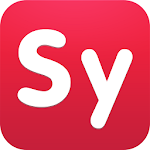ID: com.devsense.symbolab

• Author:

• Version:

Varies with device

• Update on:

## The description of Symbolab: Math Problem Solver

answer scanner solves any math problem with step-by-step solutions. Have a private math tutor in your pocket!

Symbolab has been used by over 200 million students to work through and understand more than one billion math problems and explanations.

Equations, integrals, derivatives, limits, and much more.

Symbolab Math Solver app is composed of over five hundred of Symbolab's most powerful math calculators:
Graphing Calculator
Fractions Calculator
Equation Calculator
Integral Calculator
Derivative Calculator
Limit calculator
Inequality Calculator
Trigonometry Calculator
Matrix Calculator
Functions Calculator
Series Calculator
ODE Calculator
Laplace Transform Calculator

Symbolab Math Solver & Homework Helper to solve math problems including Pre- Algebra, Algebra, Pre-Calculus, Calculus, Trigonometry, Functions, Matrix, Vectors, Geometry, and Statistics. Simply, scan a math problem with a math answer scanner to get started!

Symbolab is a global leader in education technology with over 200 million users worldwide. Symbolab is committed to helping students learn math, providing step-by-step math answers to any math problem, as well as AI-driven personalized learning, assessments, insights, and more.

--:--
--:--
--:--

## Symbolab: Math Problem Solver Varies with device APK for Android Varies with device+

 Version Varies with device for Android Varies with device+ Update on 2022-06-11 Installs 10.000.000++ File size 21.085.046 bytes Permissions view permissions What's new Bug fixes & enhancements

### FAQs

• Symbolab Calculator 4+ Download the app to experience the full set of Symbolab calculators. Symbolab Math Solver solves any math problem including Pre- Algebra, Algebra, Pre-Calculus, Calculus, Trigonometry, Functions, Matrix, Vectors, Geometry and Statistics. Symbolab solves any problem for free.
• Symbolab solves any problem for free. In order to view step-by-step solutions, you can subscribe weekly (\$1.99), monthly (\$4.99), or yearly (\$29.99).
• Symbolab - Math solver is a free app for Android published in the Teaching & Training Tools list of apps, part of Education. The company that develops Symbolab - Math solver is Symbolab. The latest version released by its developer is 8.14. ... The app is listed on our website since 2021-04-26 and was downloaded 809 times.
• Pricing structure: Free to try (\$. 99/week, \$2.49/mo.)